# A steel specimen is tested in tension. The specimen is 1.0 in. wide by 0.25 in. thick in the test region. By monitoring the load dial of the testing machine, it was found that the specimen yielded at a load of 12.5 kips and fractured at 17.5 kips.a. Determine the tensile stresses at yield and at fracture.b. Estimate how much increase in length would occur at 60% of the yield stress in a 2-in. gauge length.

Question
16 views

A steel specimen is tested in tension. The specimen is 1.0 in. wide by 0.25 in. thick in the test region. By monitoring the load dial of the testing machine, it was found that the specimen yielded at a load of 12.5 kips and fractured at 17.5 kips.
a. Determine the tensile stresses at yield and at fracture.
b. Estimate how much increase in length would occur at 60% of the yield stress in a 2-in. gauge length.

check_circle

Step 1

Given:

Width of specimen = 1 in

Thickness of specimen = 0.25 in

Also,

Modulus of Elasticity of steel, E = 29 x 103 ksi

Step 2

a)

The formula for tensile stress is given by,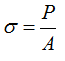Where,

A = area of cross section

The tensile stress at yield point is calculated as,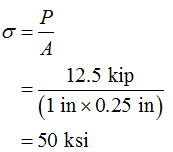The tensile stress at fracture point is calculated as,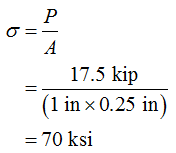Step 3

b)

Gauge length , l = 2 in

60% of yield stress is calculated as,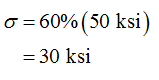The formula for modulus of elasticity is given by,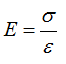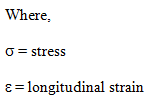The formula for longitudinal strain is given by,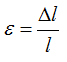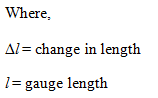The longitudinal strain is calculated as,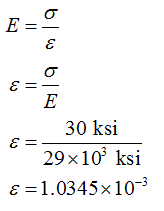The increase in length is calculated as,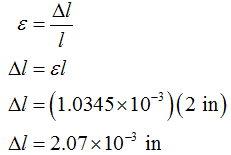...

### Want to see the full answer?

See Solution

#### Want to see this answer and more?

Solutions are written by subject experts who are available 24/7. Questions are typically answered within 1 hour.*

See Solution
*Response times may vary by subject and question.
Tagged in